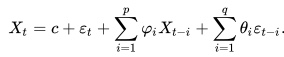# ARMA model

An ARMA model, or Autoregressive Moving Average model, is used to describe weakly stationary stochastic time series in terms of two polynomials. The first of these polynomials is for autoregression, the second for the moving average.

Often this model is referred to as the ARMA(p,q) model; where:

• p is the order of the autoregressive polynomial,
• q is the order of the moving average polynomial.

The equation is given by:Where:

• φ = the autoregressive model’s parameters,
• θ = the moving average model’s parameters.
• c = a constant,
• Σ = summation notation,
• ε = error terms (white noise).

## Difference Between an ARMA model and ARIMA

The two models share many similarities. In fact, the AR and MA components are identical, combining a general autoregressive model AR(p) and general moving average model MA(q). AR(p) makes predictions using previous values of the dependent variable. MA(q) makes predictions using the series mean and previous errors.

What sets ARMA and ARIMA apart is differencing. An ARMA model is a stationary model; If your model isn’t stationary, then you can achieve stationarity by taking a series of differences. The “I” in the ARIMA model stands for integrated; It is a measure of how many non-seasonal differences are needed to achieve stationarity. If no differencing is involved in the model, then it becomes simply an ARMA.

A model with a dth difference to fit and ARMA(p,q) model is called an ARIMA process of order (p,d,q). You can select p,d, and q with a wide range of methods, including AIC, BIC, and empirical autocorrelations (Petris, 2009).

Another, similar model is ARIMAX, which is just an ARIMA with additional explanatory variables.

## References

Petris, G. et al. (2009). Dynamic Linear Models with R. Springer Science & Business Media.

CITE THIS AS:
Stephanie Glen. "ARMA model" From StatisticsHowTo.com: Elementary Statistics for the rest of us! https://www.statisticshowto.com/arma-model/